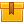# Basic Electrical Properties

## Prerequisites

Completion of the lesson, Introduction to Electricity, is recommended.

## Description

This is the second lesson in the iKNOW® Electrical Theory for Troubleshooters Library. This lesson covers Ohm's Law and the use of Ohm??s Law to calculate an unknown value. It also defines voltage, current, resistance, and power.

## Objectives

- Define voltage.
- Define current.
- Define resistance.
- Describe voltage and current relationships.
- State Ohm's Law.
- Use Ohm's Law to calculate an unknown value.
- Define power and how to use power values with Ohm's Law.

## ContentThis is the second lesson in the iKNOW™ Electrical Theory for
Troubleshooters Library. This lesson covers Ohm’s Law and the use of
Ohm’s Law to calculate an unknown value. It also defines voltage,
current, resistance, and power.

•Basic Electrical Properties

0
0 Reviews